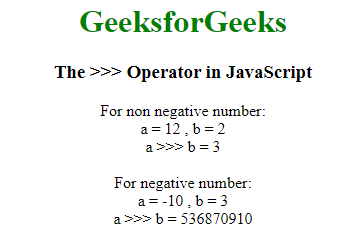# What is JavaScript >>> Operator and How to use it ?

The JavaScript >>> represents zero-fill right shift operator. It is also called the unsigned right bit shift operator. It comes under the category of Bitwise operators. Bitwise operators treat operands as 32-bit integer numbers and operate on their binary representation.

Zero-fill right shift (>>>) operator:
It is a binary operator, where the first operand specifies the number and the second operand specifies the number of bits to shift. The operator shifts the bits of the first operand by a number of bits specified by the second operand. The bits are shifted to the right and those excess bits are discarded, while 0 bit is added from left. As the sign bit becomes 0, the operator ( >>> ) returns a 32-bit non-negative integer.

Example:

```Input:
A = 6 ( 00000000000000000000000000000110 )
B = 1 ( 00000000000000000000000000000001 )

Output:
A >>> B = 3 ( 00000000000000000000000000000011 )```

Syntax:

```result = expression1 >>> expression2
```

Difference between >>> and >> :
The difference between these two is that the unsigned zero-fill right shift operator (>>>) fills with zeroes from the left, and the signed right bit shift operator (>>) fills with the sign bit from the left, thus it maintains the sign of the integer value when shifted.

Example: This example implements the use of >>> operator:

• Program:

 ` ` `<``html``> ` ` `  `<``head``> ` `    ``<``title``>>>> Operator in JavaScript ` `     `  `    ``<``style``> ` `        ``body { ` `            ``text-align: center; ` `        ``} ` `         `  `        ``h1 { ` `            ``color: green; ` `        ``} ` `    `` ` ` ` ` `  `<``body``> ` `    ``<``h1``>GeeksforGeeks ` `    ``<``h3``>The >>> Operator in JavaScript ` `     `  `    ``<``script``> ` `        ``document.write("For non negative number:<``br``>"); ` `        ``var a = 12; ` ` `  `        ``// Shift right two bits ` `        ``var b = 2; ` `        ``document.write("a = " + a + " , b = " + b); ` `        ``document.write("<``br``>a >>> b = " + (a >>> b) + '<``br``>'); ` ` `  `        ``document.write("<``br``>For negative number:<``br``>"); ` `        ``var a = -10; ` `     `  `        ``// Shift right two bits ` `        ``var b = 3;  ` `        ``document.write("a = " + a + " , b = " + b); ` `        ``document.write("<``br``>a >>> b = " + (a >>> b) + '<``br``>'); ` `    `` ` ` ` ` `  ` `

• Output:• Explanation: For non-negative numbers, zero-fill right shift (>>>) and sign-propagating right shift (>>) gives the same output. For example, 9 >>> 2 and 9 >> 2 gives same result i.e. 2. But for negative numbers, -9 >>> 2 gives 1073741821 and -9 >> 2 gives -3 as output.

```Case 1: non-negative number
12 (base 10): 00000000000000000000000000001100 (base 2)
--------------------------------
12 >>> 2 (base 10): 00000000000000000000000000000011 (base 2)
= 3 (base 10)

Case 2: negative number
-10 (base 10): 11111111111111111111111111110110 (base 2)
--------------------------------
-10 >>> 3 (base 10): 00011111111111111111111111111110 (base 2)
= 536870910 (base 10)
```My Personal Notes arrow_drop_upCheck out this Author's contributed articles.

If you like GeeksforGeeks and would like to contribute, you can also write an article using contribute.geeksforgeeks.org or mail your article to contribute@geeksforgeeks.org. See your article appearing on the GeeksforGeeks main page and help other Geeks.

Please Improve this article if you find anything incorrect by clicking on the "Improve Article" button below.